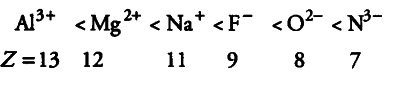# Consider the following species

Consider the following species
\${{N}^{3-}}\$, \${{O}^{2-}}\$, \${{F}^{-}}\$, \${{Na}^{+}}\$, \${{Mg}^{2+}}\$ and \${{Al}^{3+}}\$
(i) What is common in them?
(ii) Arrange them in the order of increasing ionic radii.

(i) All the given species have same number of electrons (10e-). Therefore, all are isoelectronic.
(ii) The ionic radii of isoelectronic species decreases with increase in atomic number (as magnitude of the nuclear charge increases with increase in atomic number).
Therefore, their ionic radii increase in the